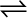7.16 What is the equilibrium concentration of each of the substances in the equilibrium when the initial concentration of ICl was 0.78 M ?

2ICl (g)I2 (g) + Cl2 (g); Kc = 0.14

The given reaction is:
Now, we can write,
Hence, at equilibrium,